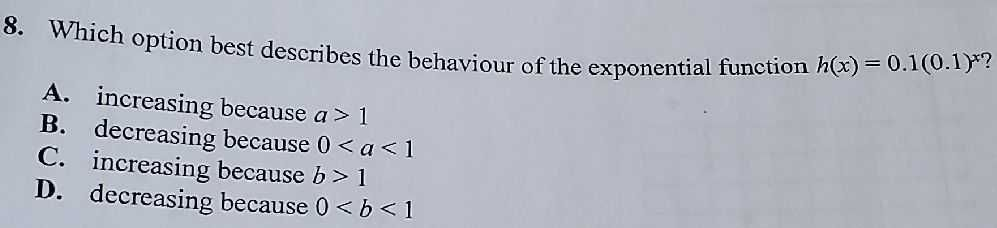### ¿Todavía tienes preguntas de matemáticas?

Pregunte a nuestros tutores expertos
Algebra
Pregunta8. Which option best describes the behaviour of the exponential function $$h ( x ) = 0.1 ( 0.1 ) ^ { x }$$ ? A. increasing because $$a > 1$$

B. decreasing because $$0 < a < 1$$

C. increasing because $$b > 1$$

D. decreasing because $$0 < b < 1$$